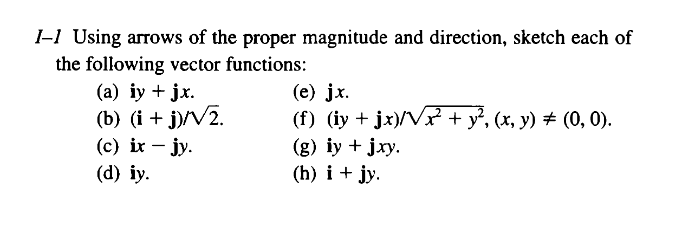# 2D Plot from Vector Function iy + jx -Div,Grad,Curl

Hi all, I am reading from this `Div, Grad, Curl and All that` by Schey

and I was wondering how to plot this with Julia(what packages needed( GR/Makie??):

this is the vector functions / the questions:I finally know how to plot all of them (thanks to J Verzani’ tutorial):

``````# https://docs.juliahub.com/CalculusWithJulia/AZHbv/0.0.7/differentiable_vector_calculus/vector_fields.html

using CalculusWithJulia
using Plots
F(x,y) = [sqrt(2), sqrt(2)] # (i+j) sqrt(2)
F(x,y) = [y, x] # (iy+jx)
F(x,y) = [x, -y] # (ix-jy)
F(x,y) = [y, 0] # (iy)
F(x,y) = [0, x] # (jx)
F(x,y) = [y, x]/sqrt(x^2+y^2) # (iy+jx)
F(x,y) = [y, x*y] # (iy+jxy)
F(x,y) = [1, y] # (i+jy)

vectorfieldplot(F, xlim=(-5,5), ylim=(-5,5), nx=10, ny=10)
``````

2 Likes
1 Like

Thanks @joa-quim it looks better